# A Smart Water Metering System Based on Image Recognition and Narrowband Internet of Things

A Smart Water Metering System Based on Image Recognition and Narrowband Internet of Things
Computer School of Yangtze University, Jingzhou 434023, China

Corresponding Author Email:
baikai@yangtzeu.edu.cn
Page:
293-298
|
DOI:
https://doi.org/10.18280/ria.330405
21 March 2019
|
Accepted:
2 July 2019
|
Published:
30 October 2019
| Citation

OPEN ACCESS

Abstract:

This paper designs a smart water metering system based on Narrowband Internet of Things (NB-IoT) and image recognition. Centering on an STM32F103ZET6 microcontroller unit (MCU), the system mainly consists of an OV7725 camera module, a secure digital (SD) card module, a liquid crystal display (LCD) module, an NB-IoT data transmission module and other peripherals. The original image of the water meter is preprocessed by graying, edge extraction, Otsu’s binarization and tilt correction. Then, the digits in the preprocessed image are recognized by a convolutional neural network (CNN) model. The effectiveness of the proposed system was verified through an experiment. Our system greatly reduces the workload and simplifies the process of water management, shedding new light on the application of information technology and the AI in water management.

Keywords:

smart water meter, narrowband internet of things (NBIoT), image processing, convolutional neural network (CNN), digit recognition

1. Introduction

Water supply companies rely on water meters to monitor the water consumption of users. The traditional water meters are read with the naked eye, which is often unstable and inaccurate. This gives rise to smart water metering systems that identify the digits on the water meter accurately based on computer vision technology.

Smart water metering techniques are constantly updated. The latest trend is to integrate remote outdoor reading with computer property management. This requires the water meter to support functions like remote transmission and centralized reading. Currently, integrated circuit (IC) card and touch memory (TM) card are suitable for prepaid smart water meters.

For subscriber identity module (SIM) card, the traditional communication methods of the general packet radio service (GPRS), namely, wireless fidelity (Wi-Fi) and Zigbee, are not suitable to provide low-cost, low-power Internet of Things (IoT) services, owing to their intense power consumption, narrow coverage and high cost.

In machine-to-machine (M2M) access, space efficiency and terminal power supply are critical. The most commonly technique for M2M access is low power wide area network (LPWAN). At present, mainstream LPWAN techniques include LoRa, SigFox and Narrowband Internet of Things (NB-IoT). These techniques enjoy many advantages, such as flexible network construction, various fast strategies, low deployment cost, and rapid commercialization.

However, LoRa and SigFox are private techniques that must be applied in a separate network, using unauthorized spectrum. Therefore, the two techniques face a certain security risk. By contrast, the NB-IoT is a standard technique of 3rd Generation Partnership Project (3GPP). It can be superimposed on the existing 2nd generation (2G)/3rd generation (3G)/Long-Term Evolution (LTE) cellular network. The frequency band of the NB-IoT is authorized, and has been adopted by operators like Vodafone, China Mobile, China Telecom and China Unicom. In general, the NB-IoT is characterized by wide coverage, multiple connections, low speed, low cost, low power consumption and excellent architecture.

This paper designs a smart water metering system based on the NB-IoT and image recognition. In the system, the digits on the water meter are recognized by a convolutional neural network (CNN) model.

The remainder of this paper is organized as follows. Section 2 reviews the relevant literature; Section 3 introduces the CNN model for digit recognition; Section 4 carries out an experiment on the proposed system and analyzes the experimental results; Section 5 puts forward the research conclusions.

2. Literature Review

Over the years, various kinds of smart water metering systems have been developed. For example, Meghdadi and Saeed  combined traditional mechanical devices with electronic water meters into a novel water metering system. The devices are low-cost, non-contact sensors that captures capacitive signals. To save power, the circuit is only powered when the server requests the measured value. The water flow is monitored by a wireless network. If water leakage occurs, the control center at the server can detect the incident through software analysis. Giurco et al.  designed a water metering IoT framework with cloud-centric storage. Under the framework, the data collected by ubiquitous sensors are processed and analyzed by a decision support interface. de Cruz et al.  proposed a reference model for an IoT middleware platform that supports intelligent IoT applications. Aloudat and Michael  developed a two-purpose water metering system based on a hydro-generator. The two purposes are sensing the water flow through adaptive processing of signals on the generated voltage, and producing electricity to charge the batteries of the smart meter. The system is embedded with a wireless transceiver to provide real-time water flow. In addition, a mobile app was designed for the users to monitor water usage. Nguyen et al.  created a smart water meter using automated meter reading (AMR) technology. The water meter enables water supply companies to provide users with clear water consumption patterns, and actively detect water leakage. Hasanvand and Berneti  presented a new multiphase flow prediction method based on neural network.

Many scholars have applied artificial intelligence (AI) technology to recognize the digits on the water meter. For instance, Liu et al.  recognized the digits on the water meter, using the Hopfield network, a recurrent neural network (RNN), and the Hemingway network. Wonders’s study  shown that by synthesising labelled training data, using a domain specific algorithm, an innovative water meter disaggregation system that uses Artificial Neural Networks, Support Vector Machine and K-Nearest Neighbour classifiers can be trained in minutes rather than hours. Considering the statistical features of orthogonal experiment, Rui et al.  selected effective features from the final feature set, and used these features to identify digit, creating an effective feature subset selection method for digit recognition. Kim et al.  proposed a real-time digit recognition method that is invariant to scale or rotation. Ma et al.  improved the digit recognition algorithm based on template matching, which greatly improves the speed of template matching in digit recognition.

3. System Design

3.1 Framework

The proposed smart water metering system is based on STM32F103ZET6 microcontroller unit (MCU). As shown in Figure 1, the system mainly consists of a first-input first-output (FIFO) OV7725 camera module, a secure digital (SD) card module, a liquid crystal display (LCD) module, an NB-IoT data transmission module and other peripherals.

## 1.png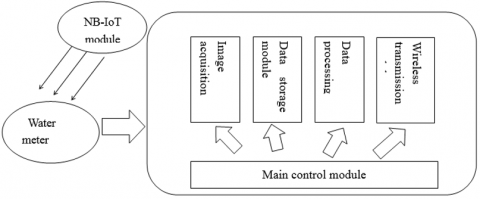Figure 1. The system framework

The working process of the proposed system can be summed up as follows: First, the mode control panel (MCP) is powered on to initialize various functional modules of the system. Next, the main control module enables the lighting device to provide illumination for the dial according to the interrupt signal of the OV7725 camera module. The camera module will collect the images of the dial, which are displayed in real time on the LCD module. The images will be stored in the SD card module. After that, the data processing module in the MCU will convert the character wheel images into digits, using image processing algorithms. Finally, the digits will be sent to the remote cloud platform through the NB-IoT wireless transmission module.

Being the cornerstone of the proposed system, the STM32F03ZET6 MCU is responsible for collecting and processing the images, and transmitting the processed results to the cloud platform. First, the MCU needs to initialize the OV7725 camera module and configure the frame interrupt signal pin. The images collected by the camera are saved to the internal memory as bitmaps. Then, the MCU runs image preprocessing algorithms like grayscale, edge detection, Otsu’s binarization and Hough transform to correct the tilt of the images. The image data are standardized into bitmaps of 20×20 pixels by the image expansion and transformation algorithm. The bitmaps are imported sequentially to the backpropagation neural network (BPNN), and the largest one is taken as the recognition result. Finally, the MCU will send the attention (AT) command through the serial port, which controls the NB-IoT module to send the recognized digits to the cloud platform.

3.2 Hardware

As shown in Figure 2, the STM32F103 MCU drives the OV7725 camera to capture the images of the character wheel in the dial of the water meter. Because the character wheel is mounted in a fixed position, the camera only needs to capture the area in the red rectangular frame on the LCD. The captured images will be saved to the SD card of the MCU in the form of bitmaps. Then, the MCU will run image processing algorithms to identify the digits in the character wheel. Finally, the MCU will send the recognition results to the cloud platform via the serial port, and the cloud platform will update its data in real time.

## 2.png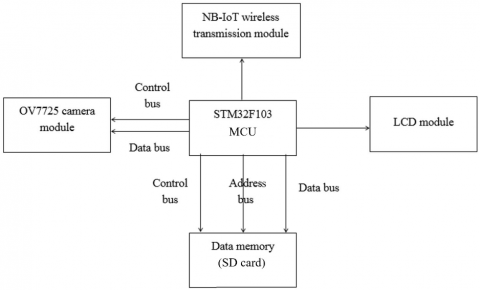Figure 2. System hardware

The MCU is a 32-bit microcontroller based on the ARM Cortex-M3 core. Compared with the commonly used Intel MCS-51, the MCU has a high performance in image processing operations. It also supports various low-power modes of operations with an 18-bit parallel camera interface. Table 1 compares the features of our MCU with those of other popular MCUs.

Table 1 compares the three series of AVR, PIC and STM32 MCUs. It is clear that the STM32F103ZET6 boasts the fastest processing speed, the lightest peripheral equipment and the lowest price. The only downside is the lack of an internal EEPROM. However, the function of the internal EEPROM is replaced with the In-Application Programming (IAP) function of flash, which is even more reliable.

The internal resources of STM32F103 include: a 64KB static random-access memory (SRAM), a 512KB flash memory, a 64MB extended SPI flash chip W25Q64 64M FALSH, 2 basic timers, 4 general-purpose timers, 2 advanced timers, 3 SPIs, 2 IIC, 5 serial ports, 1 USB, 1 CAN, 3 12-bit analog-digital converters (ADCs), 1 12-bit digital-analog converter (DAC), 1 secure digital input output (SDIO) interface, 3 light-emitting diodes (LEDs), 4 normal programmable buttons, 1 flexible static memory interface (FSMC)  interface and 112 universal input/output (IO) ports.

The system uses an OV7725 CMOS camera module (OmniVision) with FIFO cache. The camera supports the standard serial camera control bus (SCCB) interface, and is compatible with the inter-integrated circuit (I2C) bus interface. The FIFO of the camera module can temporarily store a frame of 320×240 image. With a high internal clock frequency, the camera module raises a high requirement on the MCU performance.

Table 1. Comparison of commonly used MCUs

 MCU series MEGA128 PICI8f6680 STM32F103ZET6 Number of digits 8 8 32 Pipeline technology 3-stage pipeline Dual instruction pipeline 3-stage pipeline Highest frequency 16M 40M 72M Internal flash 128k 64k 128k EEPROM 4k 1k null SPI 1 1 3 USART 2 1 5 CAN module null 1 1 USB module null null 1 Programming support C language, Assembly language, BASIC languages C language, Assembly language C language, Assembly language Suitability for C programming Extremely suitable Generally suitable Highly suitable Price (USD) 25 50 18
Note: EEPROM stands for internal electrically erasable programmable read-only memory; SPI stands for serial peripheral interface; USART stands for universal synchronous and asynchronous receiver-transmitter; CAN stands for controller area network; USB stands for universal serial bus.

The SCCB protocol defines two basic write operations, namely, a three-step write operation and a two-step write operation. The former writes data into a destination register of the slave device in three steps: the master device first transmits the slave device’s ID address and the W flag and then transmits the slave device’s destination register address, and finally writes the data. By contrast, the two-step write operation only transfers the ID address and the W flag to the slave device, as well as the address of the destination register. This operation is mainly used in conjunction with the read operation.

Due to the small memory of the MCU, the large amount of data generated in image processing must be saved into an SD card. In our system, the MCU reads and writes the communication interface of the SD card and selects the SDIO interface. The SDIO interface defines 64 commands, and the SD card can modify the values in its registers based on the commands it has received. In actual operation, the read and write operations of the SD card can be controlled by sending the corresponding command.

The main technical features of the NB-IoT include wide coverage, strong links, low power consumption and low cost. In our system, the NB-IoT technology can remotely collect real-time data, monitor equipment status and issue control command. It can also set the collection frequency and process data in time. In this way, the meter can be read more efficiently, and the leakage can be detected more accurately. Thanks to licensed spectrum organization and end-to-end security management, the NB-IoT network guarantees the anti-interference ability, data security and service quality of our system.

The USR-NB75 is developed for serial port devices and network servers to transmit data to each other through the carrier NB-IoT network. It is especially suitable for battery-powered applications. It can be implemented easily by a simple AT command. The software realizes serial-to-network bidirectional transparent data transmission in a wide coverage at a low power. If there is only one base station, the NB-IoT not only provides access to large number of devices, but also ensures a strong penetration capability and a wide range.

4. CNN-Based Digit Recognition Model

Our digit recognition model takes the structure of a classical two-stage target detection network . First, the features of the original image are extracted by a deep CNN, ResNet-101. The extracted features form a feature pyramid network (FPN), in which the features are displayed hierarchically. Then, a candidate frame was chosen from the FPN, and filtered through the region proposal network (RPN). Finally, the filtered features of the candidate frame were integrated, and the classification results, such as horizontal detection frame, tilt detection frame and image segmentation region, are generated according to the integrated features.

4.1 Feature extraction layer

The feature extraction layer is the basis of the entire deep CNN. The core of the ResNet-101  is the residual module:

$\mathrm{H}(\mathrm{y})=\mathrm{F}(y)+y$                 (1)

where, H(y) is the expected potential mapping; F(y) is the nonlinear transformation of the CNN for input y . The residual module let the network fit the residual mapping rather than fit the expected potential mapping directly.

Since the character wheel of the water meter is rather small, the digit recognition task of our model is a small target detection operation. However, the feature extraction module of ResNet-101 is a network model that generates feature map in the forward direction. The final feature map is too small (7×7) to ensure the good detection effect on small targets. To solve the problem, the FPN was introduced to enhance the detection effect of small targets, i.e. the character wheel of water meter.

Let $\mathrm{C} 2, \mathrm{C} 3, \mathrm{C} 4$ and $\mathrm{C} 5$ be the output feature maps of the last four feature extraction modules, conv2, conv3, conv4 and conv5, of ResNet- $101 .$ Then, a $1 \times 1$ convolution kernel was adopted to process $\mathrm{C} 2, \mathrm{C} 3, \mathrm{C} 4$ and $\mathrm{C} 5,$ creating feature maps $C_{21}, C_{31}, C_{41}$ and $C_{51} .$ Meanwhile, $\mathrm{C} 3, \mathrm{C} 4$ and $\mathrm{C} 5$ were up-sampled from the top to the bottom, producing feature maps $C_{32}, C_{42}$ and $C_{52} .$ Finally, $C_{21}, C_{31}$ and $C_{41}$ as well as $C_{32}, C_{42}$ and $C_{52}$ were added and combined with $C_{51}$ to form the feature maps $P_{1}, P_{2}, P_{3}$ and $P_{4}$ of the FPN feature maps.

4.2 Scale normalization layer

In the CNN, the feature maps of different layers vary in scale, and must be normalized before use. In this paper, the region-of-interest alignment (RoIAlign) algorithm  is adopted to normalize the scales of the feature maps. By this algorithm, the local feature layer of the RoI is identified; then, the rectangular RoI on the feature map is meshed into $7 \times 7$ grids; finally, the coordinates of the center point of each grid are obtained by bilinear interpolation, and taken as the coordinates of the grid. The bilinear interpolation can compute eigenvalues accurately even if the grid coordinates are not integers. The bilinear interpolation can be defined as follows.

Let $Q_{11}, Q_{12}, Q_{21}$ and $Q_{22}$ be four points in the rectangular RoI, which are arranged in the clockwise direction, and $\left(x_{1}, y_{1}\right),$ $\left(x_{1}, y_{2}\right),\left(x_{2}, y_{1}\right)$ and $\left(x_{2}, y_{2}\right)$ be the coordinates of the four points, respectively. Then, the P-value of a point in the rectangular RoI can be derived from the coordinates of the four points through linear interpolation in both $x$ and $y$ directions:

$f\left(P_{1}\right) \approx \frac{x_{2}-x}{x_{2}-x_{1}} \times f\left(Q_{11}\right)+\frac{x-x_{1}}{x_{2}-x_{1}} \times f\left(Q_{21}\right)$     (2)

$f\left(P_{2}\right) \approx \frac{x_{2}-x}{x_{2}-x_{1}} \times f\left(Q_{12}\right)+\frac{x-x_{1}}{x_{2}-x_{1}} \times f\left(Q_{22}\right)$     (3)

$f(P) \approx \frac{y_{2}-y}{y_{2}-y_{1}} \times f\left(P_{1}\right)+\frac{y-y_{1}}{y_{2}-y_{1}} \times f\left(P_{2}\right)$   (4)

Before scale normalization, the local feature layer l of the RoI must be determined based on the information (i.e. coordinates and score) of the RoI:

$l=\left|l_{0}+\log _{2}\left(\frac{\sqrt{w h}}{235}\right)\right|$               (5)

where, l0=5 is the reference value of the local feature layer; w and are the length and width of the RoI, respectively; 235 is the size of the original image imported into the CNN. Once the local feature layer is identified, the scale normalization of that layer can be implemented by the RoIAlign algorithm.

4.3 Model training and optimization

In addition to model design, the prediction effect of the CNN depends heavily on model training and optimization. The training method must be selected carefully to optimize the parameters and layers of the model, because the training is a long-term repeated iteration of the image processor.

In this paper, the stochastic gradient descent (SGD) method is employed to update the model parameters during the training process. The SGD can approximate the data distribution of the whole training set, based on the information of a few small-scale samples. To accelerate the convergence, the momentum factor was introduced to control the learning rate of model training:

$m_{t}=\varepsilon \times m_{t-1}+w_{t}$                        (6)

$\Delta \vartheta=-\sigma \times m_{t}$              (7)

where, $\mathcal{E}$ is coefficient of momentum factor; $\sigma$ is the learning rate; $w_{t}$ is the gradient signal of each error.

For the CNN model, the prediction error, i.e. the deviation of the predicted value from the truth value, can be directly measured by the loss function. The error can be minimized by the backpropagation (BP) algorithm. In our model, both the RoI layer and the prediction layer output information. Hence, a loss function was added to each of the two layers.

The RoI layer outputs two types of information: the score and the coordinates of the RoI. Thus, the loss function of this layer can be defined as:

$S\left(\left\{p_{i}\right\},\left\{t_{i}\right\}\right)=\sum_{i} s_{C}\left(p_{i}, p_{i}^{*}\right) / N_{c}+\beta \sum_{i} p_{i}^{*} S_{r}\left(t_{i}, t_{i}^{*}\right) / N_{r}$     (8)

where, $p_{i}^{*}$ is the positivity/negativity of the $\operatorname{RoI}\left(p_{i}^{*}=0 \text { or } 1\right)$ $N_{C}$ is the log function of classification loss; $N_{r}$ is the smooth function of coordinate regression loss.

5. Experiment and Results Analysis

This section aims to verify the effect of the proposed system through experiment. The specific steps of the experiment are introduced below.

5.1 Experimental procedure

To reduce the scale of the input data, the red (R), green (G) and blue (B) values of the original image were obtained by bit operation according to the 16-bit color bitmap of the RGB565 format. Then, the psychological formula was adopted to preprocess the image:

Gray$=R \times 0.299+G \times 0.587+B \times 0.114$       (9)

Then, the image was saved as an 8-bit grayscale bitmap. The original color image of the character wheel in the water meter is shown in Figure 3(a) and the image after graying is presented in Figure 3(b).

## 3.png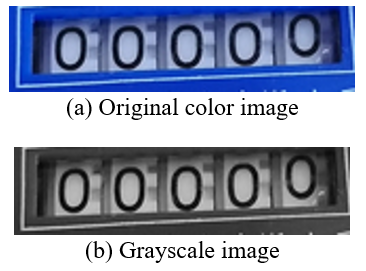Figure 3. Comparison between original image and grayscale image

To position and recognize the character wheel, the tilt of the grayscale image must be corrected. The first step is to extract the edge information from the image. Here, the Canny edge detector is adopted to extract such information through Gaussian smoothing, gradient calculation, non-maximum suppression and double threshold edge detection. Among them, non-maximum suppression compares the gradient of the current point with that in the gradient direction, and sets it to zero rather than the maximum. The double threshold edge detection involves a high threshold and a low threshold. If the gradient is above the high threshold, it will be changed to 255; if the gradient is below the low threshold, it will be changed to zero. In addition, if the gradient values of the previous and subsequent points are both lower than the high threshold, both will be set to zero. The edges extracted from the character wheel are shown in Figure 4.

## 4.pngFigure 4. Edges extracted from the character wheel

Next, binarization was adopted to eliminate the messy edges, which may otherwise affect the tilt correction. The key to binarization lies in the rationality of threshold: some unnecessary contents will be extracted if the threshold is too small, and some useful data will be lost if the threshold is too large. In this paper, the Otsu’s algorithm, the maximum inter-class variance segmentation algorithm, is employed to divide the grayscale of pixels in the image into two categories with a threshold. The greater the variance between the two categories, the better the binarization. The binarized image is displayed in Figure 5 below.

## 5.png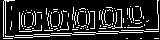Figure 5. The binarized image

On this basis, the Hough transform was introduced to detect the straight line between the upper and lower boundaries of character wheel. Then, the angle between the straight line and the horizontal axis was taken as the tilt angle. To correct the tilt, the image was rotated by the tilt angle. The rotated image is shown in Figure 6.

## 6.png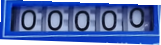Figure 6. Image after tilt correction

Next, Otsu’s binarization was performed again to identify the background of the image. The image after the removal of background information is provided in Figure 7.

## 7.png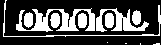Figure 7. Preprocessed image on character wheel

Based on Figure 7, a minimum rectangular area was selected to maximize the distribution of white points. The upper boundary of this area was determined in the following manner.

Firstly, a small rectangular area was defined with a certain width and height from the image center, and the number of white points in this area was counted. Then, the upper boundary was moved upward to enlarge the rectangular area, and the number of white points in the enlarged area was counted. Next, the two numbers were compared to see if the difference is greater than a threshold, which was preset to exclude noise interference. If yes, the upper boundary was moved upward again, and the other operations were repeated. Otherwise, the upper boundary was taken as the final upper boundary of the minimum rectangular area. The above steps were implemented iteratively until the difference between the said two numbers is smaller than the threshold. The lower, left and right boundaries of the minimum rectangular area were determined similarly. The final minimum rectangular area is shown in Figure 8.

## 8.png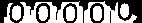Figure 8. The minimum rectangular area

Then, the character wheel image was divided by the width of each digit, for the digits are of the same width. The segmentation results are displayed in Figure 9.

## 9.png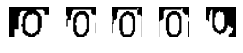Figure 9. The segmentation results

After the training, the weight and threshold matrix of the CNN were saved to a text file. The pixel data of the bitmaps were imported to the MCU as the input of the CNN. Then, the CNN performed the forward calculation of the weights and thresholds in the text file. Finally, the output layer node with the largest value was taken as the recognition result of the CNN.

In this experiment, the proposed system was applied to recognize 9,544 BMP bitmaps. The recognition rates are recorded in Table 2 below.

5.2 Analysis of experimental results

Table 2. Recognition rates of our system

 Sample numbers Digits Success rate 923 0 97.18% 612 1 98.37% 1,008 2 98.51% 925 3 98.05% 727 4 99.45% 1,068 5 98.88% 1,290 6 98.22% 903 7 97.34% 1,056 8 98.11% 1,032 9 98.35%

As shown in Table 2, the CNN model of our system achieved greater-than-97% success rate in the recognition of digits on water meter images, indicating that the CNN model can recognize the digits of water meter in an accurate manner.
6. Conclusions

Based on the cutting-edge techniques of remote wireless communication and image recognition, this paper develops a smart water metering system that uses the CNN and the NB-IoT to remotely recognize the digits on the water meter. The reading and remote transmission modules of our system can be installed on traditional water meters, eliminating the need to entirely scrap the original water meters. Therefore, the proposed system is very suitable for the upgrade of old water meters, especially those in remote rural areas. In addition, the proposed system effectively reduces the workload and simplifies the process of water management. The research results shed new light on the application of information technology and the AI in water management.

Acknowledgements

This study has been supported by the Scientific Research Projects of Hubei Provincial Department of Education (B2017032).

References

 Meghdadi, M., Saeed, G. (2011). The design and management of intelligent systems in mechanical domestic water meters. Intelligent Control and Automation, 2(4): 424-429. https://doi.org/10.4236/ica.2011.24049

 Giurco, D.P., White, S.B., Stewart, R.A. (2010). Smart metering and water end-use data: conservation benefits and privacy risks. Water, 2(3): 461-467. https://doi.org/10.3390/w2030461

 da Cruz, M.A.A., Rodrigues, J.J.P.C., Al-Muhtadi, J., Korotaev, V.V., de Albuquerque, V.H.C. (2018). A reference model for internet of things middleware. IEEE Internet of Things Journal, 5(2): 871-883. https://doi.org/10.1109/JIOT.2018.2796561

 Aloudat, A, Michael, K. (2011). Toward the regulation of ubiquitous mobile government: A case study on location-based emergency services in Australia. Electronic Commerce Research, 11(1): 31-74. https://doi.org/10.1007/s10660-010-9070-0

 Nguyen, K.A., Stewart, R.A., Zhang, H., Jones, C. (2015). Intelligent autonomous system for residential water end use classification: Autoflow. Applied Soft Computing, 31: 118-131. https://doi.org/10.1016/j.asoc.2015.03.007

 Hasanvand, M., Berneti, S.M. (2015). Predicting oil flow rate due to multiphase flow meter by using an artificial neural network. Energy Sources, Part A: Recovery, Utilization, and Environmental Effects, 37(8): 840-845. https://doi.org/10.1080/15567036.2011.590865

 Liu, L., Li, J., Zhang, Z. (2000). The Hopfield neural network model and algorithm for domination numbers of graphs. Lanzhou Railway University National Science, 19(6): 94-95.

 Wonders, M., Ghassemlooy, Z., Alamgir Hossain, M. (2016). Training with synthesised data for disaggregated event classification at the water meter. Expert Systems with Applications, 43: 15-22, https://doi.org/10.1016/j.eswa.2015.08.033

 Jin, R., Li, X., Yan, Y., Xu, M., Ku, J., Xu, S., Hu, X. (2015). Detection method of multi-target recognition of potato based on fusion of hyperspectral imaging and spectral information. Transactions of the Chinese Society of Agricultural Engineering, 31(16): 258-263. https://doi.org/10.11975/j.issn.1002-6819.2015.16.034

 Kim, S., Lee, S.H., Ro, Y.M. (2015). Image-based coin recognition using rotation-invariant region binary patterns based on gradient magnitudes. Journal of Visual Communication and Image Representation, 32: 217-223. https://doi.org/10.1016/j.jvcir.2015.08.011

 Ma, B., Bian, S.H., Huang, K., Han, Z.H., Wang, C.T. (2012). The comparison of method for numeral recognition algorithm. 2012 Proceedings of International Conference on Modelling, Identification and Control, 60(3): 272-276. https://doi.org/10.1007/s11042-011-0829-7

 Ding, X., Zhang, J., Yang, J. (2018). A robust two-stage algorithm for local community detection. Knowledge-Based Systems, 152: 188-199. https://doi.org/10.1016/j.knosys.2018.04.018

 Sun, G., Bin, S. (2018). A new opinion leaders detecting algorithm in multi-relationship online social networks. Multimedia Tools and Applications, 77(4): 4295-4307. https://doi.org/10.1007/s11042-017-4766-y

 Liang, Y., Tang, Z., Yan, M., Liu, J. (2018). Object detection based on deep learning for urine sediment examination. Biocybernetics and Biomedical Engineering, 38(3): 661-670. https://doi.org/10.1016/j.bbe.2018.05.004